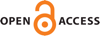Download this articleFor screen For printingRecent IssuesThe Journal About the Journal Editorial Board Editors’ Interests Subscriptions Submission Guidelines Submission Form Policies for Authors Ethics Statement ISSN: 1948-206X (e-only) ISSN: 2157-5045 (print) Author Index To Appear Other MSP JournalsGlobal regularity for the nonlinear wave equation with slightly supercritical power

### Maria Colombo and Silja Haffter

Vol. 16 (2023), No. 3, 613–642##### Abstract

We consider the defocusing nonlinear wave equation $\square u=|u{|}^{p-1}u$ in ${ℝ}^{3}×\left[0,\infty \right)$. We prove that for any initial datum with a scaling-subcritical norm bounded by ${M}_{0}$ the equation is globally well-posed for $p=5+\delta$, where $\delta \in \left(0,{\delta }_{0}\left({M}_{0}\right)\right)$.

##### Keywords
nonlinear wave equation, global regularity, supercritical equation
##### Mathematical Subject Classification 2010
Primary: 35B65, 35L15, 35L70
##### Milestones